Name:    MCAS Electric Circuits

Multiple Choice
Identify the choice that best completes the statement or answers the question.

1.

In the diagram below, the circuit has one battery and two identical light bulbs.  (MCAS 2008)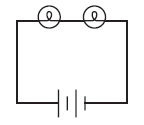If one light bulb is removed, which of the following could replace it so that the remaining light bulb is dimmer?
 a.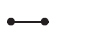b.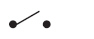c.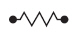d.2.

Which of the following ammeters is shown with an incorrect reading? (MCAS 2008)
 a.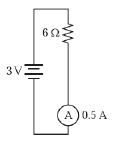b.c.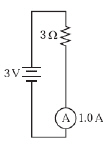d.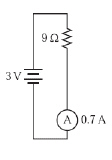3.

The diagram below shows an electrical circuit.  (MCAS 2007)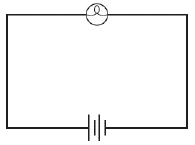Which of the following best explains why energy stored in the battery power source diminishes over time when this circuit is complete?
 a. Energy is destroyed when the light bulb operates. b. The light bulb transforms energy into light and heat. c. The light bulb converts the negative charge of electrons into light and heat. d. The power source generates energy more slowly than the light bulb consumes it.

4.

Mr. Jenkins constructed a circuit consisting of a variable source, wires, and a resistor. In order to triple the amount of current, how should he change the voltage of the source? (MCAS 2006)
 a. make the voltage three times larger c. make the voltage nine times larger b. make the voltage one-third as great d. make the voltage one-ninth as great

5.

A simple circuit is shown in the diagram below. (MCAS 2009)In which of the following circuits will bulb X have the same brightness as in the circuit above, if all batteries and all light bulbs are identical?
 a.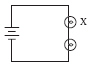b.c.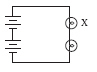d.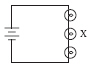6.

Large amounts of current can damage a circuit. Which of the following changes in a series circuit will result in an increase in current? (MCAS 2009)
 a. The voltage is halved and the resistance is halved. b. The voltage is halved and the resistance is doubled. c. The voltage is doubled and the resistance is halved. d. The voltage is doubled and the resistance is doubled.

7.

Based on Ohm’s law, which of the following statements explains what must happen when the voltage across a resistor is decreased? (MCAS 2009)
 a. The resistance of the resistor increases. b. The resistance of the resistor decreases. c. The current through the resistor increases. d. The current through the resistor decreases.

8.

A circuit diagram is shown below. (MCAS 2009)Which component in the diagram converts chemical energy into electrical energy?
 a. component W b. component X c. component Y d. component Z

9.

Which of the following actions would increase the current through a circuit component? (MCAS 2010)
 a. decreasing the power in the component b. increasing the resistance of the component c. increasing the voltage across the component d. placing another identical component in series in the circuit

10.

The diagram below shows a simple circuit.  (MCAS 2011)Which component is the source of energy in this circuit?
 a.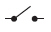b.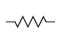c.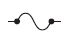d.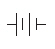11.

The measurements in the table below are from four circuits that each have the same components except for the battery. (MCAS 2011)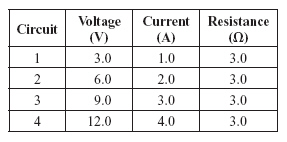Which of the following statements describes how voltage, current, and resistance are related by Ohm’s law in these circuits?
 a. As voltage increases, current decreases and resistance decreases. b. As voltage increases, current increases and resistance stays the same. c. As voltage increases, current decreases and resistance stays the same. d. As voltage increases, current stays the same and resistance decreases.

12.

The diagram below shows a simple electric circuit. (MCAS 2010)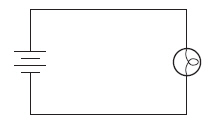Which of the following statements describes the function of the battery?
 a. It reduces the flow of electrons. b. It transmits light through the circuit. c. It pushes electrons through the circuit. d. It releases light during a chemical reaction.

13.

A battery is connected to a light bulb with copper wire to complete a circuit. The bulb immediately lights. Which of the following best describes why the bulb lights? (MCAS 2012)
 a. The battery supplies electrons,which move through the bulb but not the wire. b. The battery supplies chemicals, which pass through the bulb to the end of the wire. c. The battery supplies heat energy, which causes the bulb to produce light energy. d. The battery supplies voltage, which causes electrons throughout the circuit to move.

14.

The diagram below shows an electrical circuit with three bulbs and three switches. The switches are labeled X, Y, and Z. When all three switches are closed, as shown, all three bulbs are lit. (MCAS 2012)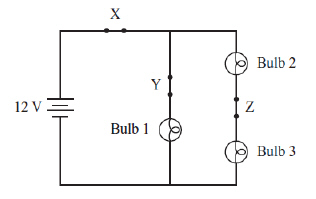Which of the following statements describes what will happen if switch Z is opened?
 a. Only bulb 1 will be lit. c. All three bulbs will be lit. b. Only bulb 3 will be lit. d. None of the bulbs will be lit.

15.

A student has a circuit that is missing a component at location X, as shown in the diagram below. (MCAS 2013)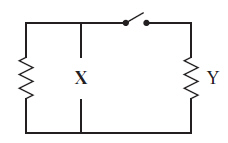The student wants component Y to warm up after the switch is closed. Which of the following components should the student add to the circuit at location X?
 a.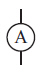b.c.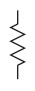d.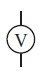Problems

16.

The graph below relates the current to voltage data for a resistor.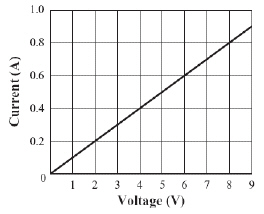Which of the following is the value of the resistor? (Modified MCAS 2007)

17.

The two resistors shown below are connected to identical power sources. Resistor 1 has a resistance of 30 W, and resistor 2 has a resistance of 45 W. The current in resistor 1 is 2 A.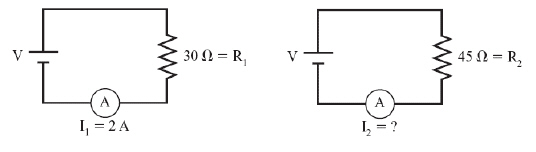What is the current in resistor 2? (Modified MCAS 2007)

18.

What is the voltage in a circuit with a current of 3 A and a total resistance of 12 W? (Modified MCAS 2006)

19.

What is the voltage across the terminals of a 23 W resistor that has 0.065 A of current flowing through it? (Modified MCAS 2010)

20.

An electric circuit is shown below. The accompanying table shows the current measured at different levels of resistance.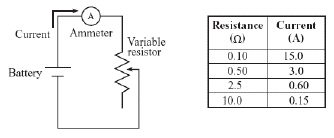Based on the data shown in the table, what is the voltage drop across the variable resistor?  (Modified MCAS 2006)

21.

A microwave oven uses 10 A of current when connected to a wall outlet that provides 120 V of electricity. What is the resistance of the microwave oven? (Modified MCAS 2010)

22.

The diagram below shows a simple circuit.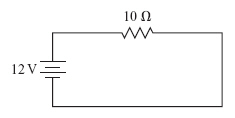What is the current in the circuit?  (Modified MCAS 2011)

23.

A simple circuit has a current of 3.0 A and a resistance of 5.0 W. What is the voltage supplied by the battery of this circuit? (Modified MCAS 2012)

24.

An appliance draws 4 A of current when connected to 120 V. What is the resistance of the appliance? (Modified MCAS 2013)

25.

A current of 2 A passes through an 8 W load. What is the potential difference across the load? (Modified MCAS 2013)

26.

The graph below shows the relationship between current and voltage in a circuit. Resistance in the circuit remains constant.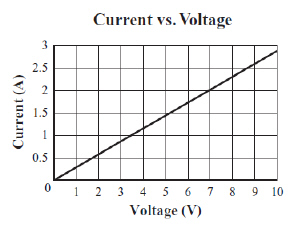What is the resistance of this circuit? (Modified MCAS 2012)

Open-Response

27.

A circuit with three identical light bulbs is shown in the diagram below. (MCAS 2008)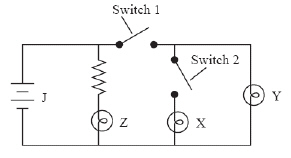a. Identify the symbol labeled J in the circuit.

b. Describe what each light bulb does in each of the following situations:

• when switches 1 and 2 are open
• when switches 1 and 2 are closed
• when switch 1 is open and switch 2 is closed
• when switch 1 is closed and switch 2 is open

28.

In an experiment, the current is varied as it goes through the resistor in a circuit. An ammeter measures the current going through the resistor and a voltmeter measures the voltage across the resistor. The data are recorded and shown below. (MCAS 2007)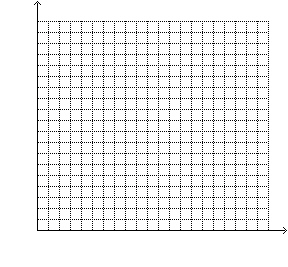a.       Using the data in the table, make a graph of the voltage versus the current in the circuit. Voltage should be on the y-axis. Make sure the graph has clearly labeled axes and a proper scale.

b.       Find the slope of the graphed line. Show your calculations and include units in your answer.

c.       Explain what the slope represents.

29.

A student performs an experiment to determine the relationships among voltage, current, and resistance. The student’s procedure includes the following steps: (MCAS 2013)
• Connect a 3.0 V battery to a 42 W resistor.
• Measure the current using an ammeter and record the value.
• Replace the 42 W resistor with a 54 W resistor, and then with a 66 W resistor, measuring and recording the current for each resistor.

The table below shows the data collected.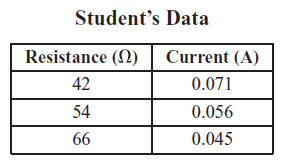a.       Draw a schematic diagram of the student’s original circuit with the 42 W resistor. Be sure to label the battery and the resistor.

b.       Describe in words the relationship between current and resistance as voltage is held constant.

The student will investigate these relationships further using a different experiment.

c.       Write a procedure the student could use to test the relationships among voltage,current, and resistance if the only materials available for use are three 3.0 V batteries,one 30 W resistor, wire, and an ammeter.

d.       Make a data table similar to the Student’s Data table above to show the expected current measurements for your procedure from part (c). Show your calculations and include units in your answer.

30.

An electrician places a variable resistor in series with an LED lamp and a 6 V battery, as shown below. (Modified MCAS 2011)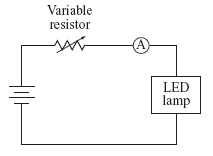LED lamps are efficient sources of light that operate at a specified voltage for a wide range of currents. The LED lamp in this circuit operates at a constant 3 V for all settings of the variable resistor, while the remaining 3 V is always dropped across the variable resistor.
a.       Calculate the current passing through the variable resistor when it is set to each of the values shown in the table below. Show your calculations and include units in your answers.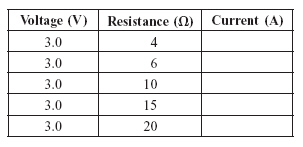b.       On the grid below, make a graph using your answers to part (a). Current should be on the y-axis and resistance should be on the x-axis. Clearly label the axes and use a proper scale.c. Based on the graph, describe what happens to the LED lamp as the resistance decreases.
The maximum recommended current for the LED lamp is 0.3 A.

d.       If the LED lamp is operated for a long time with the variable resistor set at 4W, describe the most likely effect on the LED lamp.